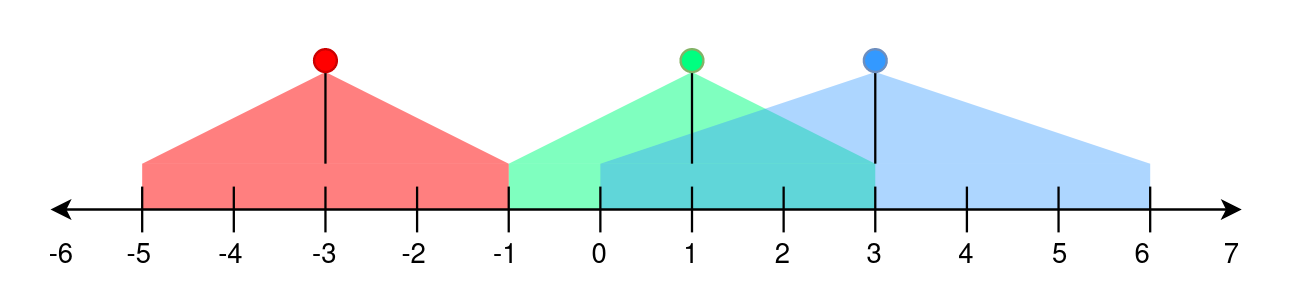# 2021 Brightest Position on Street

A perfectly straight street is represented by a number line. The street has street lamp(s) on it and is represented by a 2D integer array `lights`. Each `lights[i] = [positioni, rangei]` indicates that there is a street lamp at position positioni that lights up the area from `[positioni - rangei, positioni + rangei]` (inclusive).

The brightness of a position `p` is defined as the number of street lamp that light up the position `p`.

Given `lights`, return the brightest position on the street. If there are multiple brightest positions, return the smallest one.

Example 1:``````Input: lights = [[-3,2],[1,2],[3,3]]
Output: -1
Explanation:
The first street lamp lights up the area from [(-3) - 2, (-3) + 2] = [-5, -1].
The second street lamp lights up the area from [1 - 2, 1 + 2] = [-1, 3].
The third street lamp lights up the area from [3 - 3, 3 + 3] = [0, 6].

Position -1 has a brightness of 2, illuminated by the first and second street light.
Positions 0, 1, 2, and 3 have a brightness of 2,
illuminated by the second and third street light.
Out of all these positions, -1 is the smallest, so return it.
``````

Example 2:

``````Input: lights = [[1,0],[0,1]]
Output: 1
Explanation:
The first street lamp lights up the area from [1 - 0, 1 + 0] = [1, 1].
The second street lamp lights up the area from [0 - 1, 0 + 1] = [-1, 1].

Position 1 has a brightness of 2, illuminated by the first and second street light.
Return 1 because it is the brightest position on the street.
``````

Example 3:

``````Input: lights = [[1,2]]
Output: -1
Explanation:
The first street lamp lights up the area from [1 - 2, 1 + 2] = [-1, 3].

Positions -1, 0, 1, 2, and 3 have a brightness of 1,
illuminated by the first street light.
Out of all these positions, -1 is the smallest, so return it.
``````

Constraints:

• `1 <= lights.length <= 105`
• `lights[i].length == 2`
• `-108 <= positioni <= 108`
• `0 <= rangei <= 108`
 `````` 1 2 3 4 5 6 7 8 9 10 11 12 13 14 `````` ``````class Solution: def brightestPosition(self, lights: List[List[int]]) -> int: d = defaultdict(int) for light in lights: d[light - light] += 1 d[light + light + 1] -= 1 max_idx, max_val = -1, -1 cur = 0 for k in sorted(d.keys()): cur += d[k] if cur > max_val: max_idx = k max_val = cur return max_idx``````